Seven-segment display (SSD)

Goal: Be able to control a single and multiple SSD using microcontrollers. Understand the operation of shift registers (1. SSD powered by SEVEN wires

A Seven-segment display (SSD) unit is a simple LED package, typically all of the cathodes (negative terminals) or all of the anodes (positive terminals) of the segment LEDs are connected and brought out to a common pin; this is referred to as a "common cathode" or "common anode" device. The SSD we are going to use in this lab is a 'common cathode' unit.Fig. 1 The internal connection (schematic) of the SSD in this lab

The decimal number (1-10) in Fig. 1 are the pin numbers of the SSD unit. The letters (A-G, DP) are the corresponding LEDs.
You can map the schematic to the real SSD unit using Fig. 2, or directly check the datasheet of the SSD (LSHD5503).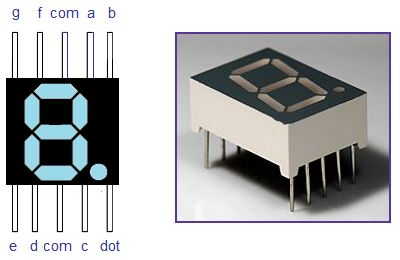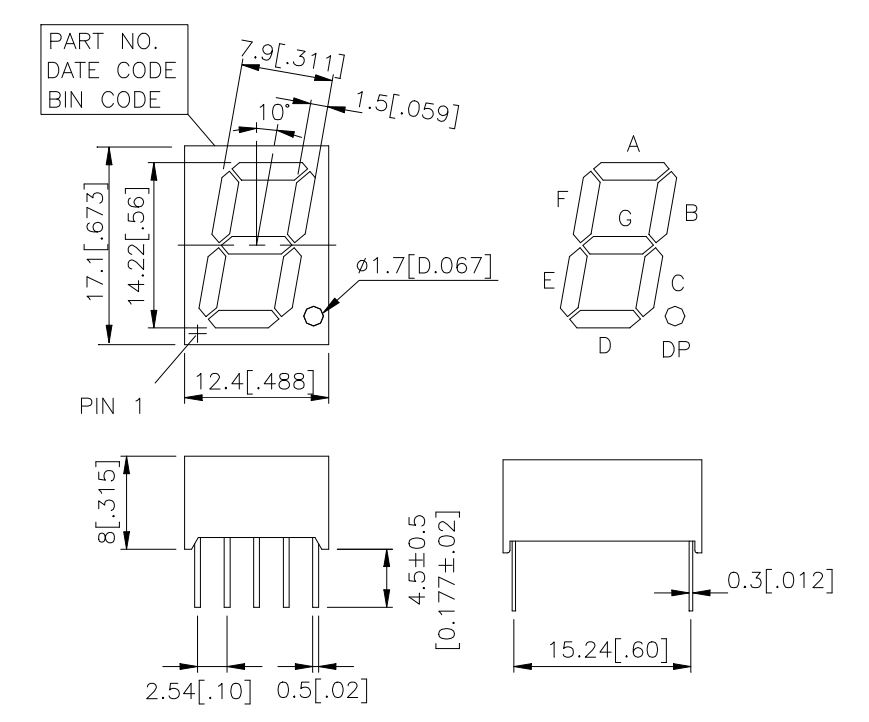Fig. 2 SSD pin/LED map.

With the LED/pin map, you can power up the corresponding LEDs and display different numbers and letters.

2. Experiments

Use the 5V voltage supply on the Arduino Board (do not go beyond that) as the power supply, connect the SSD pins to resistors to limit the current (protect the SSD unit).By powering up a certain group of LEDs, try to display numbers from 0, 1, 2, 3, ..., 9. The following figure shows you all the LEDs in the SSD were powered up: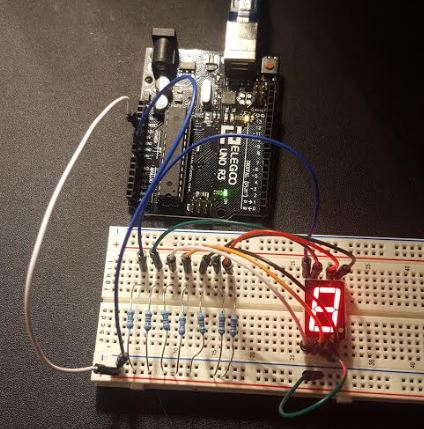The seven LEDs in the SSD device were individually controlled by SEVEN wires. I can turn off a certian combination of wires to display a number on it. Refer to the map again:To display a '6' there, I need to turn off 'B":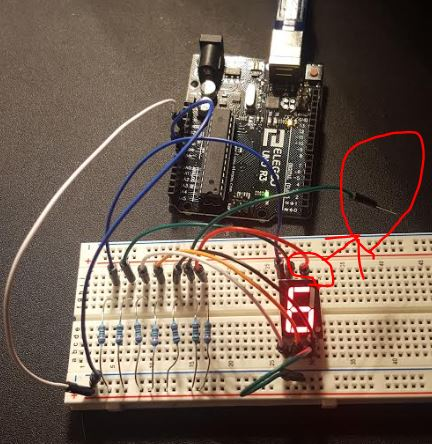See the figure above, I just plugged off one wire that used to be connected to 'B'.

Try to display all the numbers from 0 - 9.

The method in section 1 is definitely not good. It takes too much 'pin resource' just to power up one single SSD device.
In this section, we'll use a Digital Decoder to control the SSD device.

We know that 3 bits can have 2^3=8 combinations. So we can simplify the SSD control by using a 'decoder' which can convert your 3-bit input code to an 8-bit code and drive the SSD indirectly.

We are going to use M74HC4543 to drive our LED.
Connect 74HC4543 (the decoder) to your SSD:
('L' represents GND, 'H' represents 5V)Control the SSD by change the voltage at A-D of the decoder, try to display 0, 1, 2, 3, ..., 9

Now, you can create the same results but just control 4 pins instead of 7 pins. This is important because you saved a lot of pin resources, which means you can save lots of space on a real circuit board!

2.3 SSD powerd by ONE wire (only one digital line to control all seven pins)

In this section, we'll use a 'shift register' integrated circuit (IC) to receive the SSD control input SERIALLY, and then deliver all the code to the SSD device in PARALLEL.
The top view of the 'shift register' can be found below: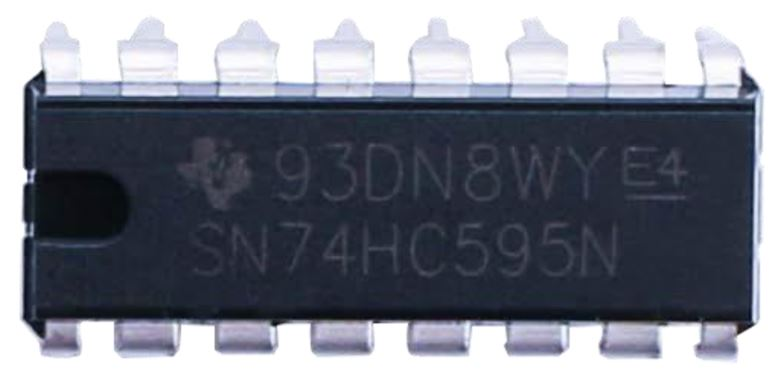74HC595N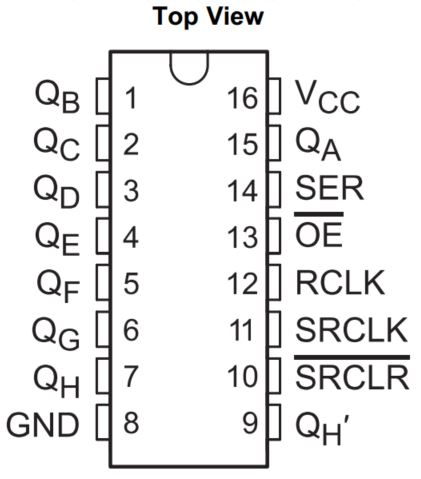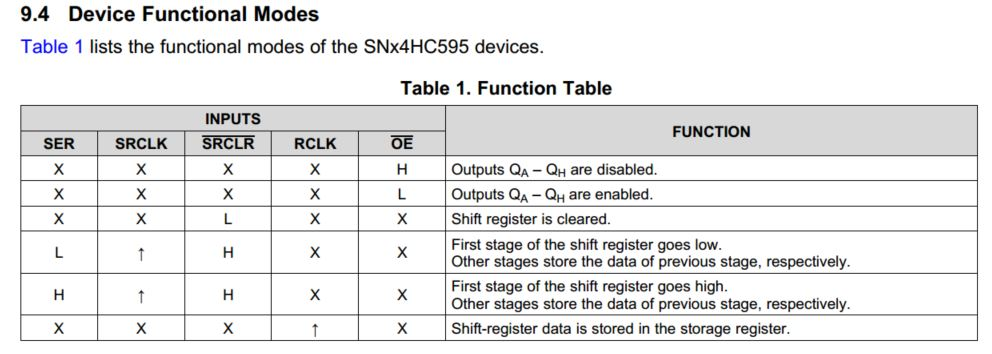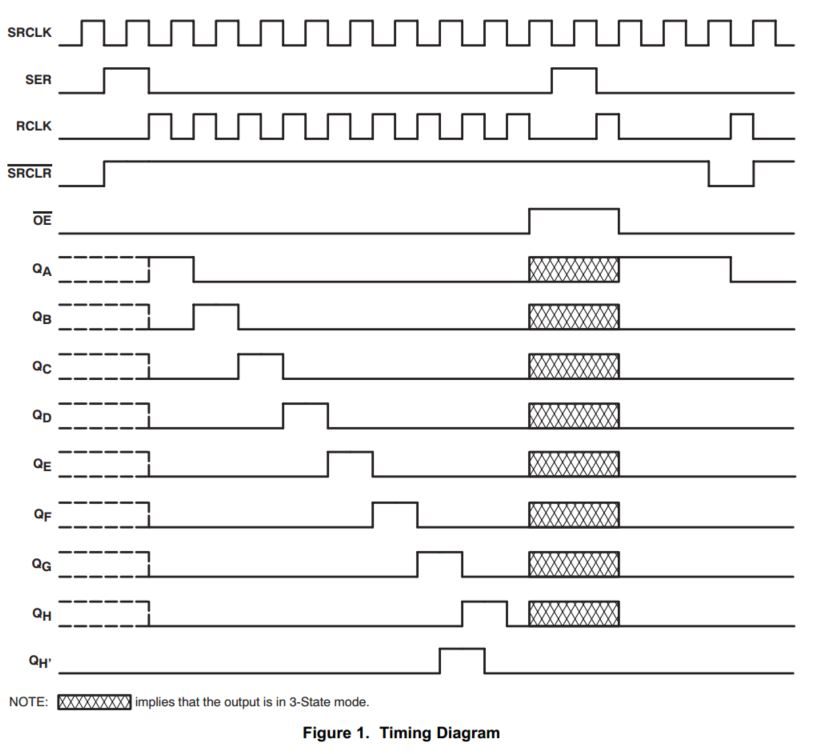From the internal circuit diagram below, we can tell that 'SER' is the one SERIALLY deliver binary data to the first stage of Register, which will be passed to the following ones. 'RCLK' seems like the 'Switch' for the Parallel output. 'SRCLK' is used to shift the data being fed into the first Register to the following ones.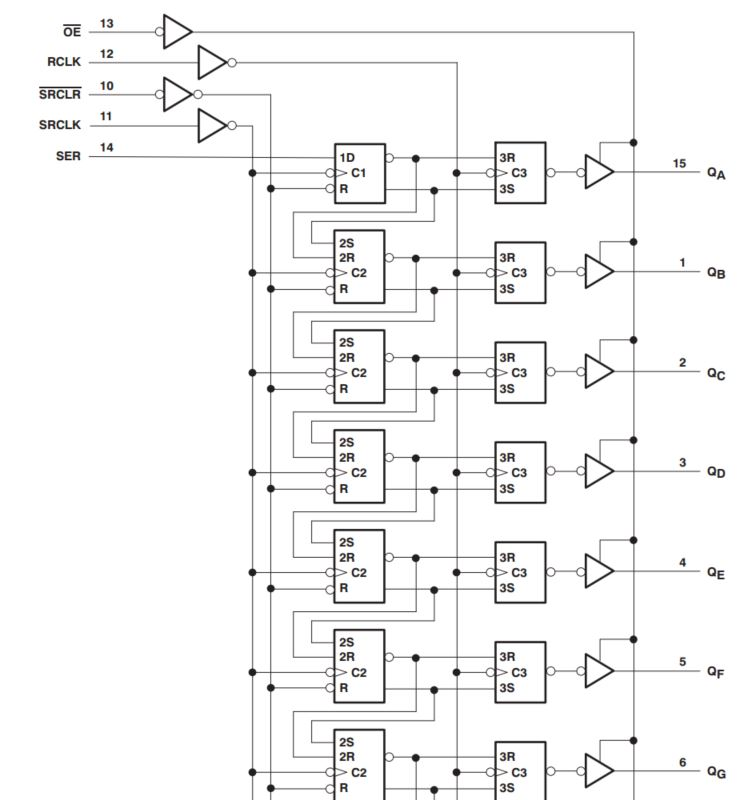The operation of the shift register may seem difficult for you if you don't have much 'digital logic' background.

Arduino has a library/function called 'shiftOut()' can manage this job for you if you don't want to handle the details of the sequential logic.

Let's test the shift register using simple LEDs first.

Make the following connection to the hardware as shown below.Use the following code: (this is the example of using LSBFIRST):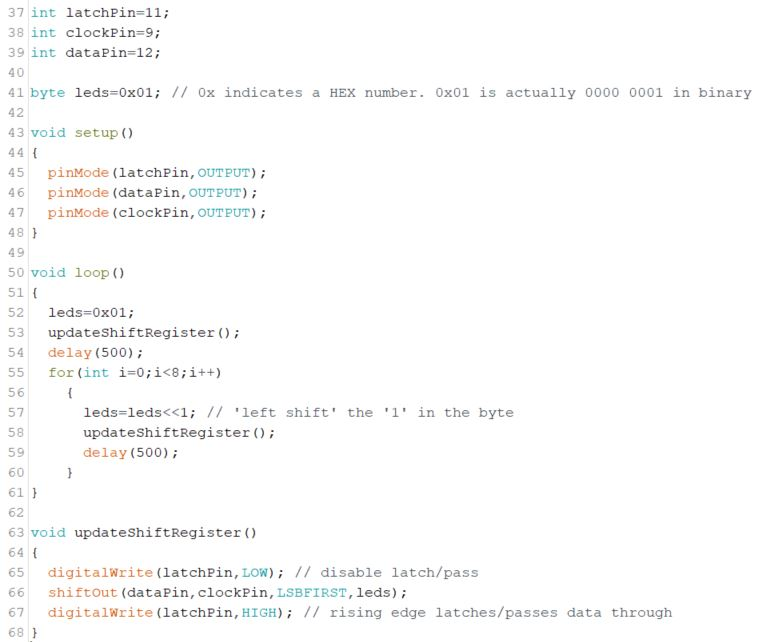The demonstration:

Make sure you understand that the shifted LEDs you saw in the video are not shifted by the 'shift refister' IC as what you saw. It is just changing it's state every half seconds. The shift register shifted the data in variable 'leds' to and latch them at the same time (in paralllel) to the out put of the shift regfister: Q0 - Q7.

It might be confusing that you are shifting the '1' in byte variable 'leds'. This is done by an internal shift function inside the Arduino CPU. So there are three things being 'shifted' but only the command 'shiftOut(dataPin,clockPin,LSBFIRST,leds)' is shifted by the 'shift refister' IC we used here.

Now, we know how to use the 74HC595N chip. There is only one data line 'SER' instead of three or more. This is the strategy being used in real-life. We can use this single 'shift register' IC to control more than ONE SSD device, and even make a digital clock out of it.

Let's display decimal numbers to an SSD device using 74HC595N first (refer to the schematic in the example above to wire up your cricuit) and use the following code (please note the changes made in this code):

// SSD, single, counts, MSBFIRST, revised 9/16/2020
int latchPin=11;// RCLK
int clockPin=9;// SRCLK
int dataPin=12;//SER

byte SSDs=0x3F; // in binary, it is 0011 1111, which only turns off G and H, will display 0

void setup()
{
pinMode(latchPin,OUTPUT);
pinMode(dataPin,OUTPUT);
pinMode(clockPin,OUTPUT);
}

void loop()
{
SSDs=0x06; //1
delay(500);
SSDs=0x5B; //2
delay(500);
SSDs=0x4F; //3
delay(500);
SSDs=0x66; //4
delay(500);
SSDs=0x6D; //5
delay(500);
SSDs=0x7D; //6
delay(500);
SSDs=0x07; //7
delay(500);
SSDs=0x7F; //8
delay(500);
SSDs=0x6F; //9
delay(500);
SSDs=0x3F; //0
delay(500);
}

{
digitalWrite(latchPin,LOW); // disable latch/pass
shiftOut(dataPin,clockPin,MSBFIRST,SSDs);
digitalWrite(latchPin,HIGH); // rising edge latches/passes data through
}

The 'delay(500)' will generate half second delay to detect if there is any change in the SSD code. However, we don't have any changes in this code, it is static, so the number on the SSD will not be changed.

If you want to change it over time, we should modify the code. For example, let's make this counts from 0 to 9 and then back to 0.

Demonstration:

Of course you can make the delay shorter to roll it faster.

Repeat this example first and then change it to LSBFIRST and modify the code accordingly and demonstrate it in a video.

2.4 SSD powerd by ONE wire (More than ONE SSD devices)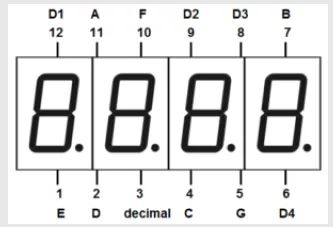All the cathodes are connected together.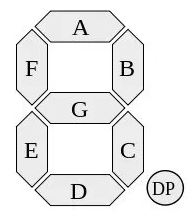All the 4 decimal digits are sharing the same A-G and DP. Every single decimal digit can be enabled/disabled by turning on/off D1-D4.

If we enable/disable everyone very quickly, your eye can't resolve the change and it looks static. So we can display 4 different decimal numbers at the 4 digits.

Let's try to display '2019' to the 4 digits.

Connect DIG1 - DIG4 to pin7 - pin4 on your Arduino board through 4 resistors.(The Arduino code is not provided)